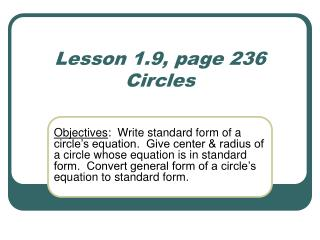DownloadDownload PresentationLesson 1.9, page 236 Circles

# Lesson 1.9, page 236 Circles

Download Presentation## Lesson 1.9, page 236 Circles

- - - - - - - - - - - - - - - - - - - - - - - - - - - E N D - - - - - - - - - - - - - - - - - - - - - - - - - - -
##### Presentation Transcript

1. Lesson 1.9, page 236Circles Objectives: Write standard form of a circle’s equation. Give center & radius of a circle whose equation is in standard form. Convert general form of a circle’s equation to standard form.

2. Definition • Circle: the set of all points in a plane that are at a fixed distance form a fixed point, the center, in the plane • Standard Form for Equation of a Circle (x – h)2 + (y – k)2 = r2 where (h,k) is the center of the circle and r is the radius.

3. Equation of Circle with Center at the Origin

4. See Example 3, page 236 • Check Point 3: Write the standard form of the equation of the circle with center (0,0) and radius 4.

5. Circles not Centered at (0,0) • A circle centered at (-2,5) with a radius=7 has what equation? • A circle whose equation is has what as its center and radius? center = (2,-6) and radius=2

6. See Example 4, page 237 • Check Point 4: Write the standard form of the equation of the circle with center (5, -6) and radius 10.

7. See Example 5, page 237 • Check Point 5: a) Find the center and radius of the circle whose equation is (x + 3)2 + (y – 1)2 = 4.

8. y 10 x -10 10 -10 (x + 3)2 + (y – 1)2 = 4 • b) Use results from part (a) to graph the equation. C) Use the graph to determine the domain and range.

9. Converting General Form toStandard Form • Standard Form is achieved by Completing the Square. (a + b)2 = a2 + 2ab + b2

10. Example: Watch and listen. • x2 + y2 – 2x – 6y = 2

11. Review: Completing the Square • Find the value of c that makes x2 + 12x + c a perfect square. Then write the trinomial as a perfect square. • Think: What is half of b? • Square this number to find c. • Try this one: Find the value of c that makes x2 - 16x + c a perfect square. Then write the trinomial as a perfect square.

12. Steps for Completing the Square 1) Re-write equation in (x2 + x) + (y2 + y) = r2 form. (Move any constants to the right side.) 2) Find half the coefficient of x. Square this number. Add this to both sides of the equation. 3) Find half the coefficient of y. Square this number. Add this to both sides of the equation. 4) Re-write the left hand side in (x – h)2 + (y – k)2form; simplify the right side. 5) Find the center (h,k) and radius, r.

13. y 10 x -10 10 -10 See Example 6, page 238 • Check Point 6: Write in standard form and graph: x2 + y2 + 4x – 4y – 1 = 0.

14. Extra Example